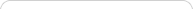##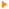Teaching with Sketchpad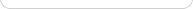•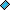- plays a video
•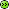- opens a web page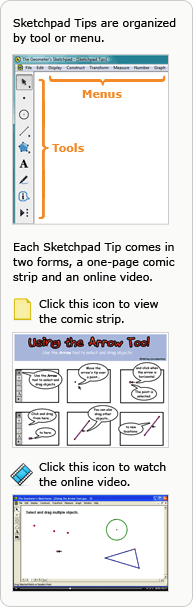Sketchpad Tips give you a quick overview of Sketchpad's tools and menu commands. Click the categories below to open the lists and immediately view the comic strips or short online videos.

###Tools

• Select, deselect, and drag objects with the Arrow tool
• Select and drag multiple objects with the Arrow tool
• Test a construction using the Drag Test
• Rotate objects with the Arrow tool
• Dilate objects with the Arrow tool
• Construct a point with the Point tool
• Construct a point on an object with the Point tool
• Construct a circle with the Compass tool
• Construct a segment with the Straightedge tool
• Construct a ray with the Straightedge tool
• Construct a line with the Straightedge tool
• Construct a polygon with the Polygon tool
• Construct a polygon and its segment edges with the Polygon tool
• Construct only the segment edges of a polygon with the Polygon tool
• Label an object with the Text tool
• Change an object's label with the Text tool
• Move an object's label with the Text tool
• Type a caption with the Text tool
• Insert an object's label into a caption
• Insert a dynamic value into a caption
• Make a tick mark on a path with the Marker tool
• Make an angle marker with the Marker tool
• Change the number of strokes of a tick mark or angle marker with the Marker tool
• Drag a tick mark with the Marker tool
• Draw with the Marker tool
• Measure an angle using its angle marker
• Insert the name of an angle into a caption using its angle marker
• Change the properties of an angle marker using Edit | Properties
• Change the type or direction of an angle marker using Edit | Properties | Marker
• Click an object with the Information tool to show its description

###Custom Tools

• Create a custom tool by pressing the Custom tool icon and choosing Create New Tool
• Choose a custom tool from the Custom Tools menu
• Use a custom tool from a different document
• Copy a custom tool from one document to another
• Find and use a custom tool using Help | Sample Sketches & Tools
• Save a tool in the Tool folder
• Use a tool from the Tool folder

###File

• Open, print, and save a document
• Add or remove pages using File | Document Options
• Rename pages using File | Document Options
• Rearrange pages using File | Document Options
• Change to a different page using page tabs
• Create a Link button using Edit | Action Buttons | Link

###Edit

• Undo actions using Edit | Undo
• Redo actions you have undone, using Edit | Redo
• Copy an object using Edit | Copy
• Paste an object using Edit | Paste
• Paste an image into Sketchpad using Edit | Paste Picture
• Copy from Sketchpad to paste into another program
• Create a Hide/Show button using Edit | Action Buttons | Hide/Show
• Create a Movement button using Edit | Action Buttons | Movement
• Create a Presentation button using Edit | Action Buttons | Presentation
• Select all objects by choosing the Arrow tool and then using Edit | Select All
• Select all points by choosing the Point tool and then using Edit | Select All Points
• Select all circles by choosing the Compass tool and then using Edit | Select All Circles
• Select all segments by choosing the Segment tool and then using Edit | Select All Segments
• Drag a picture into your sketch
• Resize a picture with the Arrow tool
• Crop a picture to a polygon
• Make a picture translucent using Edit | Properties | Opacity
• Change the layer of a picture using the Context menu
• Merge a point to a path using Edit | Merge
• Split a point from a path using Edit | Split
• Merge two points using Edit | Merge Points
• View and change an object's properties using Edit | Properties
• Change the number of decimal places in a measurement using Edit | Properties
• Change Sketchpad's settings using Edit | Preferences
• Make objects and text bigger and easier to see
• Make points easier to select using Edit | Preferences | Tools

###Display

• Change a line’s width or pattern using Display | Line Style
• Change a point’s size using Display | Point Style
• Color an object using Display | Color
• Color a label using Display | Color
• Color a caption or measurement using Display | Color
• Delete an object using Edit | Clear
• Delete an object using the Delete or Backspace key
• Hide an object using Display | Hide
• Show hidden objects using Display | Show All Hidden
• Show one hidden object only using Display | Show All Hidden
• Trace an object using Display | Trace
• Erase traces using Display | Erase Traces
• Animate an object using Display | Animate
• Show the Motion Controller using Display | Show Motion Controller
• Create an Animation button using Edit | Action Buttons | Animation
• Format text using the Text Palette
• Insert mathematical symbols into captions using the Text Palette

###Construct

• Construct a midpoint using Construct | Midpoint
• Construct an intersection using Construct | Intersection
• Construct a point on an object using Construct |Point on Object
• Construct a segment using Construct | Segment
• Construct a ray using Construct | Ray
• Construct a line using Construct | Line
• Construct a parallel line using Construct | Parallel Line
• Construct a perpendicular line using Construct | Perpendicular Line
• Construct an angle bisector using Construct | Angle Bisector
• Construct a circle using Construct | Circle by Center+Point
• Construct a circle using Construct | Circle by Center+Radius
• Construct an arc using Construct | Arc through 3 Points
• Construct an arc using Construct | Arc on Circle
• Construct an arc interior using Construct | Arc Interior | Arc Sector
• Construct an arc interior using Construct | Arc Interior | Arc Segment
• Construct a polygon interior using Construct | Interior
• Construct a circle interior using Construct | Interior
• Construct a locus using Construct | Locus

###Transform

• Mark a vector using Transform | Mark Vector
• Translate an object using Transform | Translate
• Mark a point as a center of rotation using Transform | Mark Center
• Rotate an object using Transform | Rotate
• Mark a point as a center of dilation using Transform | Mark Center
• Dilate an object using Transform | Dilate
• Mark a straight object as a mirror using Transform | Mark Mirror
• Reflect an object using Transform | Reflect
• Mark an angle using Transform | Mark Angle
• Rotate an object by a marked angle using Transform | Rotate
• Mark a distance using Transform | Mark Distance
• Translate an object by a marked distance using Transform | Translate
• Mark a ratio of two segments using Transform | Mark Ratio
• Mark a ratio of three points using Transform | Mark Ratio
• Dilate an object by a marked ratio using Transform | Dilate
• Mark a scale factor using Transform | Mark Scale Factor
• Dilate an object by a marked scale factor using Transform | Dilate
• Measure a ratio of two segments using Measure | Ratio
• Measure a ratio of three points using Measure | Ratio
• Iterate a construction using Transform | Iterate
• Iterate a construction to a selected depth by holding the Shift key and choosing Transform | Iterate to Depth
• Construct the terminal point of an iteration using Transform | Terminal Point
• Define a transformation by selecting two points and using Transform | Define Custom Transform
• Use a custom transformation by choosing its name from the Transform menu

###Measure

• Measure the length of a segment using Measure | Length
• Measure a distance using Measure | Distance
• Measure an angle by selecting three points and using Measure | Angle
• Measure an angle by making a selection rectangle around its vertex and choosing Measure | Angle
• Measure an area using Measure | Area
• Measure a perimeter using Measure | Perimeter
• Measure a circumference using Measure | Circumference
• Measure an arc angle using Measure | Arc Angle
• Measure an arc length using Measure | Arc Length
• Measure the coordinates of a point using Measure | Coordinates
• Measure the x-coordinate of a point using Measure | Abscissa
• Measure the y-coordinate of a point using Measure | Ordinate
• Change the number of decimal places in measured coordinates using Edit | Preferences
• Measure the slope of a line using Measure | Slope
• Find the equation of a line or circle using Measure | Equation
• Create a slider using Measure | Value of Point

###Number

• Create a new number (parameter) using Number | New Parameter
• Change the value of a number (parameter)
• Animate a parameter using Display | Animate Parameter
• Calculate an expression using Number | Calculate
• Edit a calculation by double-clicking with the Arrow tool
• Click a value in the sketch to enter it into the Calculator
• Enter a value or function into the Calculator
• Understand the precision of a measurement
• Create a table using Number | Tabulate
• Add a row to a table by double-clicking with the Arrow tool
• Remove a row from a table using Number| Remove Table Data
• Plot data points using Graph | Plot Table Data
• Create a function using Number | New Function
• Edit a function by double-clicking with the Arrow tool

###Graph

• Create a coordinate system using Graph | Show Grid
• Change the scale of a coordinate system
• Move a coordinate system
• Make points snap to nearby locations using Graph | Snap Points
• Show or hide grid lines using Graph | Show/Hide Grid
• Display a trigonometric axis using Graph | Grid Form
• Change the color of grid lines using Display | Color
• Plot points using Graph | Plot Points
• Plot a point by selecting two values and using Graph | Plot as (x,y)
• Plot a function using Graph | Plot New Function
• Plot a function using Graph | Plot Function
• Construct a geoboard

NOTE: If you are using Internet Explorer and there is a security warning bar across the top of this window, click it and choose Allow Blocked Content. If you are unable to download the .zip file, right-click the download link and choose Save Target As to save to your computer.